# Using Measured Parameters in a Constraint Satisfaction ComputationThis task explains how to use parameters valuated by a formula in the Constraints Satisfaction Editor. In the scenario detailed below, you want to calculate the length of a pad, which is part of a bottle cap to reach a given volume.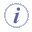• Knowledgeware parameters that are valuated by a formula are now taken into account by the constraints satisfaction function. For a formula to be taken into account:
• Access the Formula Editor and write a knowledge formula: Volume.1 = smartVolume( Pad.1) for example.
• Open the Constraint Satisfaction Editor and enter the following constraint formula:
 ```Volume.1 == 1L; Pad.1\Height > 0mm;`````` Pad.1\Height < 10000mm```
• It is possible to find more than one solution to a single problem (See Step 9).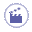1. Open the KwoCap.CATPart File. The following image is displayed.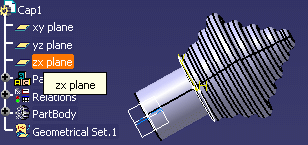1. Create a parameter that will compute the cap volume.

• Click the Formula icon (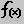).
• In the scrolling list, select the Volume parameter and click New Parameter of type.
• In the Edit name or value of the current parameter field, edit the name of the volume parameter: Cap_Volume in this scenario.
• Click Add Formula and enter the following formula:
 ```Cap_Volume = Volume_Pad1 + Volume_Pad2 + Volume_Pad3 + Volume_Pad4 + Volume_Pad5 + Volume_Pad6 + Volume_Pad7 + Volume_Pad8 + Volume_Pad9```
• Click OK twice to validate.
2. From the Start > Knowledgeware menu, access the Product Engineering Optimizer workbench.

3. Click the Constraint Satisfaction icon (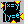). Click OK in the opening window. The ConstraintSatisfaction.1 window opens.

4. Enter the following formula into the editor:

 ```Cap_Volume == 0.2L; PartBody\Pad.1\FirstLimit\Length > 2mm; PartBody\Pad.1\FirstLimit\Length < 100mm; PartBody\Pad.2\FirstLimit\Length > 2mm; PartBody\Pad.2\FirstLimit\Length < 50mm```
1. Click the Parse arrow (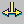). For more information about the interface, see Getting Familiar with the Constraints Satisfaction Editor.

2. Click the Results tab. In the Number of solutions to be found field, enter 4.

3. Click Solve. Click OK in the Solving was successful dialog box.

4. In the lower part of the editor, select line 3 and click Apply solution. The solution is applied to the parameters.

5. Click Apply: The model is updated. Click OK to exit the Constraint Satisfaction editor. Click No when prompted to save the log.